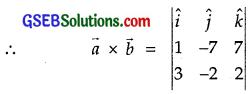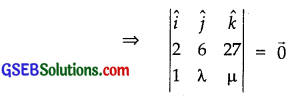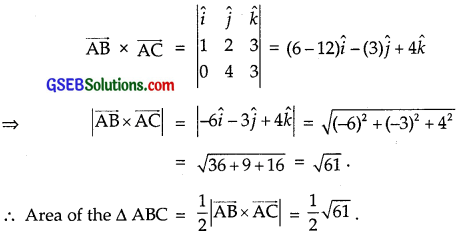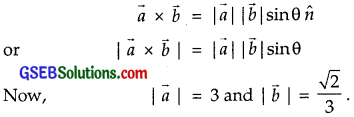# GSEB Solutions Class 12 Maths Chapter 10 Vector Algebra Ex 10.4

Gujarat Board GSEB Textbook Solutions Class 12 Maths Chapter 10 Vector Algebra Ex 10.4 Textbook Questions and Answers.

## Gujarat Board Textbook Solutions Class 12 Maths Chapter 10 Vector Algebra Ex 10.4

Question 1.
Find |$$\vec{a}$$ × $$\vec{b}$$|, if $$\vec{a}$$ = $$\hat {i}$$ – 7$$\hat {j}$$ + 7$$\hat {k}$$
and $$\vec{b}$$ = 3$$\hat {i}$$ – 2$$\hat {j}$$ + 2$$\hat {k}$$.= (- 14 + 14)$$\hat {i}$$ – (2 – 21)$$\hat {j}$$ + (- 2 + 21)$$\hat {k}$$
= – (- 19)$$\hat {j}$$ + 19$$\hat {k}$$ = 19$$\hat {j}$$ + 19$$\hat {k}$$
|$$\vec{a}$$ × $$\vec{b}$$| = $$\sqrt{(19)^{2}+(19)^{2}}$$ = 19$$\sqrt{1+1}$$ = 19$$\sqrt{2}$$.Question 2.
Find a unit vector perpendicular to each of the vectors $$\vec{a}$$ + $$\vec{b}$$ and $$\vec{a}$$ – $$\vec{b}$$, where
$$\vec{a}$$ = 3$$\hat {i}$$ + 2$$\hat {j}$$ + 2$$\hat {k}$$ and $$\vec{b}$$ = $$\hat {i}$$ + 2$$\hat {j}$$ – 2$$\hat {k}$$.
Solution:
We have:
$$\vec{a}$$ = 3$$\hat {i}$$ + 2$$\hat {j}$$ + 2$$\hat {k}$$ and $$\vec{b}$$ = $$\hat {i}$$ + 2$$\hat {j}$$ – 2$$\hat {k}$$.
∴ $$\vec{a}$$ + $$\vec{b}$$ = (3 + 1)$$\hat {i}$$ – (2 + 2)$$\hat {j}$$ + (2 – 2)$$\hat {k}$$
= 4$$\hat {i}$$ + 4$$\hat {j}$$
∴ $$\vec{a}$$ – $$\vec{b}$$ = (3 – 1)$$\hat {i}$$ + (2 – 2)$$\hat {j}$$ + (2 + 2)$$\hat {k}$$
= 2$$\hat {i}$$ + 4$$\hat {k}$$
Now, ($$\vec{a}$$ + $$\vec{b}$$) × ($$\vec{a}$$ – $$\vec{b}$$)= (16 – 0)$$\hat {i}$$ – (16 – 0)$$\hat {j}$$ + (0 – 8)$$\hat {k}$$
= 16$$\hat {i}$$ – 16$$\hat {j}$$ – 8$$\hat {k}$$.
∴ A unit vector perpendicular to both the vectors ($$\vec{a}$$ + $$\vec{b}$$) and ($$\vec{a}$$ – $$\vec{b}$$) is given byQuestion 3.
If a unit vector $$\vec{a}$$ makes angle $$\frac{π}{3}$$ with $$\hat {i}$$, $$\frac{π}{4}$$ with $$\hat {j}$$
and an acute angle θ with $$\hat {k}$$, then find θ and hence the components of $$\vec{a}$$.
Solution:
Let $$\vec{a}$$ = a1$$\hat {i}$$ + a2$$\hat {j}$$ + a3$$\hat {k}$$
such that |a1$$\hat {i}$$ + a2$$\hat {j}$$ + a3$$\hat {k}$$| = 1.
As per question:
(a1$$\hat {i}$$ + a2$$\hat {j}$$ + a3$$\hat {j}$$).$$\hat {i}$$ = |$$\vec{a}$$| |$$\hat {i}$$|cos $$\frac{π}{3}$$
⇒ (a1) (1) = (1)(1) × $$\frac{1}{2}$$
⇒ a1 = $$\frac{1}{2}$$
Also (a1$$\hat {i}$$ + a2$$\hat {j}$$ + a3$$\hat {k}$$). $$\hat {j}$$
= |$$\vec{a}$$| |$$\hat {i}$$| cos $$\frac{π}{3}$$
⇒ (a1)(1) = (1)(1) × $$\frac{1}{\sqrt{2}}$$ ⇒ a2 = $$\frac{1}{\sqrt{2}}$$.
As |$$\vec{a}$$| = 1, therefore $$a_{1}^{2}+a_{2}^{2}+a_{3}^{2}$$ = 1Question 4.
Show that ($$\vec{a}$$ – $$\vec{b}$$) × ($$\vec{a}$$ + $$\vec{b}$$) = 2($$\vec{a}$$ × $$\vec{b}$$).
Solution:
($$\vec{a}$$ – $$\vec{b}$$) × ($$\vec{a}$$ + $$\vec{b}$$)
= $$\vec{a}$$ × $$\vec{a}$$ + $$\vec{a}$$ × $$\vec{b}$$ – $$\vec{b}$$ × $$\vec{a}$$ – $$\vec{b}$$ × $$\vec{b}$$
= $$\vec{a}$$ × $$\vec{b}$$ + $$\vec{a}$$ × $$\vec{b}$$ = 2($$\vec{a}$$ × $$\vec{b}$$).
[∵ $$\vec{a}$$ × $$\vec{a}$$ = $$\vec{b}$$ × $$\vec{b}$$ = $$\vec{0}$$
and – $$\vec{b}$$ × $$\vec{a}$$ = $$\vec{b}$$ × $$\vec{b}$$]

Question 5.
Find λ and µ, if (2$$\hat {i}$$ + 6$$\hat {j}$$ + 27$$\hat {k}$$) × ($$\hat {i}$$ + λ$$\hat {j}$$ + µ$$\hat {k}$$) = $$\vec{0}$$
Solution:
(2$$\hat {i}$$ + 6$$\hat {j}$$ + 27$$\hat {k}$$) × ($$\hat {i}$$ + λ$$\hat {j}$$ + µ$$\hat {k}$$) = $$\vec{0}$$⇒ (6µ – 27λ)$$\hat {i}$$ – (2µ – 27)$$\hat {j}$$ + (2λ – 6)$$\hat {k}$$ = $$\vec{0}$$
Comparing, we have:
6µ – 27λ = 0 ………………….. (1)
2µ – 17 = 0 ⇒ µ = $$\frac{27}{2}$$ …………………. (2)
and 2λ – 6 = 0 ⇒ λ = $$\frac{6}{2}$$ = 3 …………………. (3)
Putting for λ, µ in (1), We see that it is satisfied as
6($$\frac{27}{2}$$) – 27(3) = 81 – 81 = 0
Hence, λ = 3 and µ = $$\frac{27}{2}$$.Question 6.
Given that $$\vec{a}$$.$$\vec{b}$$ = 0 and $$\vec{a}$$ × $$\vec{b}$$. What can you conclude about the vectors a and b?
Solution:
Given $$\vec{a}$$.$$\vec{b}$$ = 0 and $$\vec{a}$$ × $$\vec{b}$$ = $$\vec{0}$$.
⇒ ($$\vec{a}$$ = 0 or $$\vec{b}$$ = $$\vec{0}$$ or $$\vec{a}$$ ⊥$$\vec{b}$$) and ($$\vec{a}$$ = $$\vec{0}$$ or $$\vec{b}$$ = $$\vec{0}$$ or $$\vec{a}$$ || $$\vec{b}$$)
⇒ Either $$\vec{a}$$ = 0 or $$\vec{b}$$ = $$\vec{0}$$
⇒ [∵ $$\vec{a}$$ ⊥ $$\vec{b}$$ and $$\vec{a}$$ || $$\vec{b}$$ can never hold simultaneously.

Question 7.
Let the vectors $$\vec{a}$$, $$\vec{b}$$ and $$\vec{c}$$ are given as a1$$\hat {i}$$ + a2$$\hat {j}$$ + a3$$\hat {k}$$,
b1$$\hat {i}$$ + b2$$\hat {j}$$ + b3$$\hat {k}$$ and c1$$\hat {i}$$ + c2$$\hat {j}$$ + c3$$\hat {k}$$.
Thus, show that $$\vec{a}$$ × ($$\vec{b}$$ + $$\vec{c}$$) = $$\vec{a}$$ × $$\vec{b}$$ + $$\vec{a}$$ × $$\vec{c}$$.
Solution:
$$\vec{b}$$ + $$\vec{c}$$ = (b1$$\hat {i}$$ + b2$$\hat {j}$$ + b3$$\hat {k}$$) + (c1$$\hat {i}$$ + c2$$\hat {j}$$ + c3$$\hat {k}$$)
= (b1 + c1)$$\hat {i}$$ + (b2 + c2)$$\hat {j}$$ + (b3 + c3)$$\hat {k}$$From (1) and (2), we conclude that
$$\vec{a}$$ × ($$\vec{b}$$ + $$\vec{c}$$) = $$\vec{a}$$ × $$\vec{b}$$ + $$\vec{a}$$ × $$\vec{c}$$.Question 8.
If either $$\vec{a}$$ = $$\vec{0}$$ or $$\vec{b}$$ = $$\vec{0}$$, then $$\vec{a}$$ × $$\vec{b}$$ = $$\vec{0}$$. Is the converse true?
Solution:
We have:
$$\vec{a}$$ = $$\vec{0}$$ ⇒ |$$\vec{a}$$| = 0
∴ $$\vec{a}$$ × $$\vec{b}$$ = |$$\vec{a}$$| |$$\vec{b}$$| sin θ $$\hat {n}$$,
where θ is the angle between $$\vec{a}$$ and $$\vec{b}$$.
= 0 × |$$\vec{b}$$| sin θ = 0
Similarly, when $$\vec{b}$$ = $$\vec{0}$$ ⇒ |$$\vec{b}$$| = 0, $$\vec{a}$$ × $$\vec{b}$$ = 0
Converse : Let $$\vec{a}$$ = a1$$\hat {i}$$ + a2$$\hat {j}$$ + a3$$\hat {k}$$
and $$\vec{b}$$ = pa1$$\hat {i}$$ + pa2$$\hat {j}$$ + pa3$$\hat {k}$$.
i.e. $$\vec{a}$$ and $$\vec{b}$$ are parallel, So θ = 0.
and |$$\vec{a}$$| ≠ 0, |$$\vec{b}$$| ≠ 0.
But $$\vec{a}$$ × $$\vec{b}$$ = |$$\vec{a}$$| |$$\vec{b}$$| sin θ $$\hat {n}$$ = 0
Thus, $$\vec{a}$$ × $$\vec{b}$$ = 0, even when |$$\vec{a}$$| ≠ 0, |$$\vec{b}$$| ≠ 0.

Question 9.
Find the area of the traiangle with vertices A(1, 1, 2), B(2, 3, 5) and C(1, 5, 3).
Solution:
The position vectors of vertices of ∆ ABC are (1, 1, 2), (2, 3, 5) and (1, 5, 5).
∴ $$\overrightarrow{\mathrm{AB}}$$ = $$\overrightarrow{\mathrm{OB}}$$ – $$\overrightarrow{\mathrm{OA}}$$
= (2$$\hat {i}$$ + 3$$\hat {j}$$ + 5$$\hat {k}$$) – ($$\hat {i}$$ + $$\hat {j}$$ + 2$$\hat {k}$$)
= $$\hat {i}$$ + 2$$\hat {j}$$ + 3$$\hat {k}$$.
$$\overrightarrow{\mathrm{AC}}$$ = $$\overrightarrow{\mathrm{OC}}$$ – $$\overrightarrow{\mathrm{OA}}$$ = ($$\hat {i}$$ + 5$$\hat {j}$$ + 5$$\hat {k}$$) – ($$\hat {i}$$ + $$\hat {j}$$ + 2$$\hat {k}$$)
= 0$$\hat {i}$$ + 4$$\hat {j}$$ + 3$$\hat {k}$$ = 4$$\hat {j}$$ + 3$$\hat {k}$$.Question 10.
Find the area of parallelogram whose adjacent sides are determined by the vectors $$\vec{a}$$ = $$\hat {i}$$ – $$\hat {j}$$ + 3$$\hat {k}$$
and $$\vec{b}$$ = 2$$\hat {i}$$ – 7$$\hat {j}$$ + $$\hat {k}$$.
Solution:Area of the parallelogram whose sides are vectors $$\vec{a}$$ and $$\vec{b}$$Question 11.
Let the vectors $$\vec{a}$$ and $$\vec{b}$$ are such that |$$\vec{a}$$| = 3 and |$$\vec{b}$$| = $$\frac{\sqrt{2}}{3}$$, then $$\vec{a}$$ × $$\vec{b}$$ is a unit vector, if the angle between $$\vec{a}$$ and $$\vec{b}$$ is
(A) $$\frac{π}{6}$$
(B) $$\frac{π}{4}$$
(C) $$\frac{π}{3}$$
(D) $$\frac{π}{2}$$
Solution:Also, |$$\vec{a}$$ × $$\vec{b}$$| is a unit vector.∴ Part (B) is the correct answer.Question 12.
Area of rectangle having vertices A, B, C and D with position vectors
(- $$\hat {i}$$ + $$\frac{1}{2}$$$$\hat {j}$$ + 4$$\hat {k}$$), ($$\hat {i}$$ + $$\frac{1}{2}$$$$\hat {j}$$ + 4$$\hat {k}$$), ($$\hat {i}$$ – $$\frac{1}{2}$$ $$\hat {j}$$ + 4$$\hat {k}$$)
and (- $$\hat {i}$$ – $$\frac{1}{2}$$$$\hat {j}$$ + 4$$\hat {k}$$) is
Solution:
The position vectors of A and B are – $$\hat {i}$$ + $$\frac{1}{2}$$$$\hat {j}$$ + 4$$\hat {k}$$
and $$\hat {i}$$ + $$\frac{1}{2}$$$$\hat {j}$$ + 4$$\hat {k}$$.
∴ $$\overrightarrow{\mathrm{AB}}$$ = [1 – (- 1)]$$\hat {i}$$ + 0.$$\hat {j}$$ + 0.$$\hat {k}$$
∴ |$$\overrightarrow{\mathrm{AB}}$$| = 2
The postion vectors of A and D are – $$\hat {i}$$ + $$\frac{1}{2}$$$$\hat {j}$$ + 4$$\hat {k}$$ and – $$\hat {i}$$ – $$\frac{1}{2}$$$$\hat {j}$$ + 4$$\hat {k}$$.
∴ |$$\overrightarrow{\mathrm{AD}}$$| = (- $$\hat {i}$$ + $$\hat {i}$$) + (- $$\frac{1}{2}$$ – $$\frac{1}{2}$$) $$\hat {j}$$ + 4$$\hat {k}$$.
∴ |$$\overrightarrow{\mathrm{AD}}$$| = 1.
Area of rectangle ABCD = |$$\overrightarrow{\mathrm{AB}}$$| |$$\overrightarrow{\mathrm{AD}}$$| = 2 × 1 = 2.
∴ Part (C) is the correct answer.